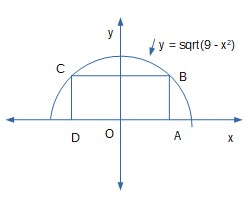# A rectangle is bounded by the x-axis and the semicircle y = square root{9-x^2}. What length and...

## Question:

A rectangle is bounded by the x-axis and the semicircle y ={eq}\sqrt{9-x^2}{/eq}. What length and width should the rectangle have so that its area is maximum?

## Critical Number of a Function:

First we need to understand the critical number to solve this problem:

Basically the maximum and minimum values of a function occur only at critical numbers but a function may or may not have maximum/minimum values at a critical number. So, First we need to understand the critical number to solve this problem.

Definition of critical number: The numbers x where {eq}f'(x) = 0 {/eq} or {eq}f'(x) {/eq} is undefined but {eq}f(x) {/eq} is defined that number x is called the critical number of {eq}f(x) {/eq}.

Given:

{eq}y = \sqrt{9 - x^2} \text{ and x-axis } {/eq}

First we will sketch the graph.Let {eq}OA = x {/eq}, then {eq}AB = y {/eq}, so the length of the rectangle is {eq}l = 2 x {/eq} and breadth of the rectangle is {eq}b = y {/eq}.

First we will compute the area of the rectangle.

As we know the formula for the area of the rectangle is:

\begin{align*} \displaystyle A &= l \times b \\ &= 2 x \times y \\ &= 2 x \times \sqrt{9 - x^2} &\text{(Plugging in the value of } y \text{)}\\ A &= x \sqrt{9 - x^2} \\ A^2 &= 4 x^2 (9 - x^2) &\text{(Squaring both sides )}\\ A^2 &= 4 ( 9 x^2 - x^4) \end{align*}

We will compute the derivative of {eq}A^2 {/eq} to get the critical point.

Take the derivative of {eq}A^2 {/eq} with respect to {eq}x {/eq}:

\begin{align*} \displaystyle \frac{d A^2}{dx} &= \frac{d}{dx} \left [4 ( 9 x^2 - x^4) \right ] \\ &= 4 ( 9 \times 2 x - 4 x^3 ) &\text{(Differentiating using the formula } \frac{d}{dx}[x^n] = n x^{n - 1} \text{)}\\ &= 4 (18 x - 4 x^3)\\ \end{align*}

Plug in {eq}\displaystyle \frac{d A^2}{dx} = 0 {/eq} for getting the critical point:

\begin{align*} \displaystyle 4 (18 x - 4 x^3) &= 0\\ - 8 x (2 x^2 - 9) &= 0 \\ - 8 x &= 0 \text{ or } 2 x^2 - 9 = 0 \\ 2 x^2 - 9 = 0 \text{ or } &\text{(} x = 0 \text{ is not possible } \\ 2 x^2 &= 9 \\ x^2 &= \frac{9}{2} \\ x^2 &= 4.5 \\ x &= \pm \sqrt{4.5} &\text{(Taking square root both sides)}\\ x &= \pm \sqrt{4.5} \\ x &= \sqrt{4.5} &\text{( } x = - \sqrt{4.5} \text{ is not possible because the length is not negative)}\\ \end{align*}

Now we will compute the value of {eq}y {/eq} at {eq}x = \sqrt{4.5} {/eq}

Plug in {eq}x = \sqrt{4.5} {/eq} in the given equation:

\begin{align*} \displaystyle y &= \sqrt{9 - (\sqrt{4.5})^2} \\ y &= \sqrt{9 - 4.5} \\ y &= \sqrt{4.5} \end{align*}

Thus, the length of the rectangle is: {eq}l = 2 x = 2 \sqrt{4.5} {/eq} and the breadth of the rectangle is: {eq}b = y = \sqrt{4.5} {/eq}.